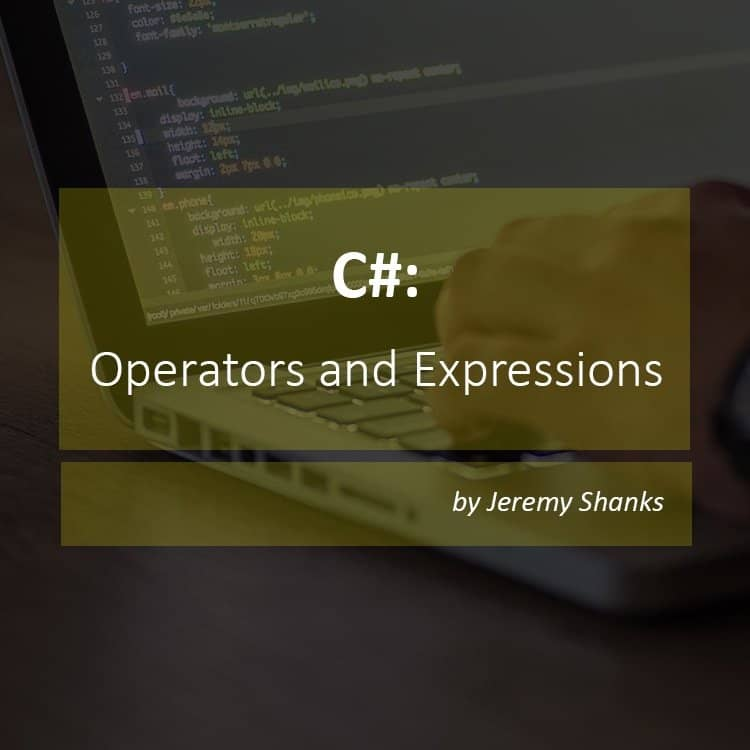# C#: Operators and ExpressionsIt’s time to get to get out your math hat because today we will be covering C# operators and expressions. In this article, I will cover the different types of operators along with how to use them properly by providing real code examples. I will also discuss expressions and how they relate to operators. This post is just one of many posts covering various topics in C# as I continue towards my goal of learning the C# programming language. I hope if you are reading this you will find some value in the content.

## Operators

Operators are nothing more than symbols that represent operations to conduct on operands. Let’s look at an example:

1 + 3

In the above example, the operator is the plus symbol which tells the CLR to add 1 and 3 together and get a value. The operands are the 1 and 3.

#### Arithmetic

Operator Description Example Result
+ Addition 1 + 5 6
Subtraction 5 – 1 4
* Multiplication 2 * 4 8
/ Division 8 / 4 2
% Modulus (Remainder) 9 % 4 1

#### Relational

Operator Description Example Result
== Is equal to  1 == 2  false
!= Is NOT equal to  1 != 2  true
> Is greater than  1 > 2  false
< Is less than  1 < 2  true
>= Is greater than or equal to  1 >= 2  false
<= Is less than or equal to  1 <= 2  true

#### Logical

Operator Description Example Result
&&  Logical AND  true && false  false
||  Logical OR true || false  true
!  Logical NOT  !false  true

#### Assignment

Operator Description Example Result
=  Equals  x = 5  x is now equal to 5
+=  Incremental Addition  x = 5
x += 2
x is now equal to 7
-=  Incremental Decrement x = 5
x += 2
x is now equal to 3
*=  Multipy by  x = 5
x *= 2
x is now equal to 10
/= Divide by  x = 8
x /= 2
x is now equal to 4
%=  Modulus  x = 3
x %= 2
x is now equal to 1

## Expressions

An expression is simply a series of one or more operands and one or more operators that can be evaluated to a single value. In writing, this probably does not make any sense so lets see an example.

In the example above i is evaluated to have the value of 3. The numbers 1 and 2 are the operands, and the plus sign(+) is the operator.

C# expressions follow the mathematics order of operations so be sure to keep that in mind when writing your expressions.

In this article, we covered the basics of operators and expressions in C#. Now you can get started writing the next big calculator application. As always if you have any questions or comment,s please leave a comment below and I will be sure to get back to you as soon as possible.